Next: Nonrelativistic Limit of the Up: Dirac Equation Previous: Dirac Particle at Rest

# Electromagnetic Interaction

We introduce the coupling to an electromagnetic field to obtain an interpretation of the internal structure of Dirac particles. Consider the interaction of a point charge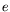with an external electromagnetic field. The 4-potential is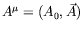, where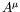is a function of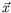only. We make the usual gauge invariant minimal substitution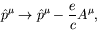(5.20)

whereis the magnitude of the charge of an electron. The Dirac equation becomes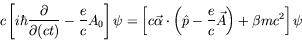(5.21)

or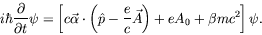(5.22)

This equation contains the interaction with the electromagnetic field: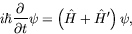(5.23)

where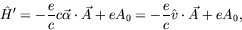(5.24)

and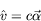(5.25)

is the classical correspondence of the relativistic velocity operator. We can see this by looking at the relativistic extension of the Ehrenfest relation: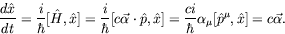(5.26)Next: Nonrelativistic Limit of the Up: Dirac Equation Previous: Dirac Particle at Rest
Douglas M. Gingrich (gingrich@ ualberta.ca)
2004-03-18